# SBI PO Prelims Quantitative Aptitude Questions 2019 (Day-35)

Dear Aspirants, Our IBPS Guide team is providing new series of Quantitative Aptitude Questions for SBI PO 2019 so the aspirants can practice it on a daily basis. These questions are framed by our skilled experts after understanding your needs thoroughly. Aspirants can practice these new series questions daily to familiarize with the exact exam pattern and make your preparation effective.

[WpProQuiz 6161]

### Click Here for SBI PO Pre 2019 High-Quality Mocks Exactly on SBI Standard

1) Train A crosses a pole in ___ seconds and Train B crosses the same pole in ___ seconds. The length of Train A is half the length of Train B. The ratio of the speed of Train A to Train B is 4: 5.

Which of the following option satisfies the two blanks given in the questions?

a) 20, 60

b) 30, 40

c) 25, 40

d) 25, 60

e) None of these

2) The profit earned when the article is sold for Rs._____ is double the profit earned when the same article is sold for Rs. 502. The cost price of the article is Rs. 420.

Which of the following option satisfies the blank given in the questions?

a) 584

b) 472

c) 546

d) 618

e) None of these

Directions (3 – 5): The questions below are based on the given Series-I. The series-I satisfy a certain pattern, follow the same pattern in Series-II and answer the questions given below.

3)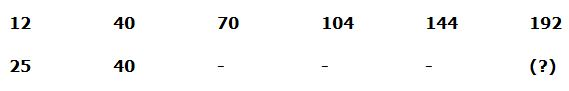a) 220

b) 160

c) 180

d) 140

e) 230

4)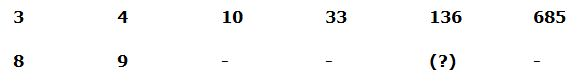a) 284

b) 256

c) 268

d) 312

e) 330

5)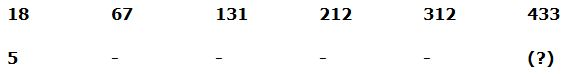a) 420

b) 382

c) 456

d) 378

e) 404

Directions (Q. 6 – 10): Study the following information carefully and answer the given questions.

There are 3 different countries (India, Canada and England) won the certain number of medals in 3 common wealth games. Most of the medals were won by England in all the years. The ratio of total number of medals won by those 3 countries in the year 2010, 2014 and 2018 is 35: 25: 32.  75 % of the medals won by those 3 countries in the year 2018 are 240. The total medals won by England in 2010 are 6 more than 44 % of total medals won by those 3 countries in 2010. In the year 2014, the difference between the medals won by England and Canada is 20. In the year 2018, total medals won by England are 20 less than the half of the medals won by all those 3 countries in the year 2018. The ratio of medals won by Canada and India in 2018 is 5: 7.

6) Find the difference between the total medals won by India in the year 2010 to that of total medals won by Canada in the year 2018, if the ratio of total number of medals won by India and Canada in the year 2010 is 7: 12?

a) 15

b) 20

c) 10

d) 5

e) None of these

7)  Total medals won by England in the year 2010 is approximately what percentage of total medals won by Canada in the year 2014, if in the year 2014, 20 % of the medals won by India?

a) 178 %

b) 162 %

c) 145 %

d) 130 %

e) 196 %

8) Find the ratio between the total medals won by India in the year 2018 to that of total medals won by those 3 countries in the year 2014?

a) 33 : 59

b) 8 : 23

c) 21 : 50

d) 17 : 42

e) None of these

9) Find the average number of medals won by Canada in all the given years together, if the total medals won by India in the year 2010 is 70 and the total medals won by England in the year in 2014 is 110?

a) 105

b) 95

c) 80

d) 130

e) 120

10) Total medals won by those 3 countries in the year 2010 is approximately what percentage more/less than the total medals won by those 3 countries in the year 2014?

a) 25 % less

b) 40 % less

c) 50 % more

d) 40 % more

e) 25 % more

The length of Train A = (1/2)* The length of Train B

The length of Train A: The length of Train B = 1: 2 (x, 2x)

Option (a),

Speed of Train A = x/20

Speed of Train B = 2x/60

Required ratio = (x/20): (2x/60) = 3: 2

Option (a) doesn’t satisfy the given condition.

Option (b),

Speed of Train A = x/30

Speed of Train B = 2x/40

Required ratio = (x/30): (2x/40) = 2: 3

Option (b) doesn’t satisfy the given condition.

Option (c),

Speed of Train A = x/25

Speed of Train B = 2x/40

Required ratio = (x/25): (2x/40) = 4: 5

Option (c) satisfies the given condition.

Option (d),

Speed of Train A = x/25

Speed of Train B = 2x/60

Required ratio = (x/25): (2x/60) = 6: 5

Option (d) doesn’t satisfy the given condition.

Option (a)

According to the question,

SP1 – CP = 2*(SP2 – CP)

584 – 420 = 2*(502 – 420)

164 = 164

Option (a) satisfies the given condition.

Option (b)

SP1 is less than SP2. So,

Option (b) doesn’t satisfy the given condition.

Option (c)

546 – 420 = 2*(502 – 420)

126 = 2*82

126 ≠ 164

Option (c) doesn’t satisfy the given condition.

Option (d)

618 – 420 = 2*(502 – 420)

198 ≠ 164

Option (d) doesn’t satisfy the given condition.

Or

CP = 420

Profit when SP = 502

=> 502 – 420 = 82

Double the profit = 82 * 2 = 164

New SP = 420 + 164 = 584

Directions (3 – 5):

Series I pattern: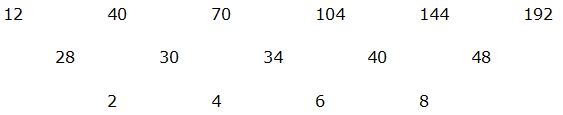The difference of difference is, 2, 4, 6, 8,…

Series II pattern: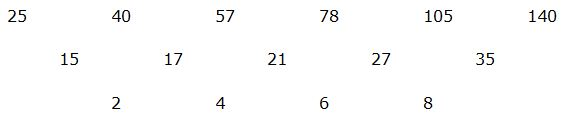Series I pattern:

3*1 + 1 = 4

4*2 + 2 = 10

10*3 + 3 = 33

33*4 + 4 = 136

136*5 + 5 = 685

Series II pattern:

8*1 + 1 = 9

9*2 + 2 = 20

20*3 + 3 = 63

63*4 + 4 = 256

256*5 + 5 = 1285

Series I pattern:

18 + 72 = 67

67 + 82 = 131

131 + 92 = 212

212 + 102 = 312

312 + 112 = 433

Series II pattern:

5 + 72 = 54

54 + 82 = 118

118 + 92 = 199

199 + 102 = 299

299 + 112 = 420

Directions (Q. 6 – 10):

The ratio of total number of medals won by those 3 countries in the year 2010, 2014 and 2018 = 35: 25: 32

75 % of the medals won by those 3 countries in the year 2018 = 240

Total medals won by those 3 countries in the year 2018 = 240*(100/75) = 320

Total medals won by those 3 countries in the year 2010 = 35*(320/32) = 350

Total medals won by those 3 countries in the year 2014 = 25*(320/32) = 250

The total medals won by England in 2010

= > 44 % of total medals won by those 3 countries in 2010 + 6

= > (44/100)*350 + 6 = 160

The difference between the medals won by England and Canada in 2014 = 20

Eng – Can = 20

Total medals won by England in the year 2018 = (320/2) – 20 = 140

The ratio of medals won by Canada and India in 2018 = 5 : 7

Total medals won by Canada and India in 2018 = 320 – 140 = 180

Total medals won by Canada in the year 2018 = (180/12)*5 = 75

Total medals won by India in the year 2018 = (180/12)*7 = 105

The ratio of total number of medals won by India and Canada in the year 2010 = 7 : 12

The total number of medals won by India and Canada in the year 2010

= > 350 – 160 = 190

The total medals won by India in the year 2010 = (190/19)*7 = 70

The total medals won by Canada in the year 2018 = 75

Required difference = 75 – 70 = 5

Total medals won by England in the year 2010 = 160

The difference between the medals won by England and Canada in 2014 = 20

Eng – Can = 20 –> (1)

Total medals won by India in the year 2014 = 250*(20/100) = 50

Total medals won by Canada and England in the year 2014 = 250 – 50 = 200

Eng + Can = 200 –> (2)

By solving the equation (1) and (2), we get,

Eng = 110, Can = 90

Total medals won by Canada in the year 2014 = 90

Required % = (160/90)*100 = 177.77 % = 178 %

The total medals won by India in the year 2018 = 105

Total medals won by those 3 countries in the year 2014 = 250

Required ratio = 105 : 250 = 21 : 50

The total medals won by India in the year 2010 = 70

The total medals won by Canada in the year 2010 = 350 – (160 + 70) = 120

The total medals won by England in the year in 2014 = 110

The total medals won by Canada in the year 2014 = 110 – 20 = 90

The average number of medals won by Canada in all the given years together

= > (120 + 90 + 75)/3 = 285/3 = 95

Total medals won by those 3 countries in the year 2010 = 350

Total medals won by those 3 countries in the year 2014 = 250

Required % = [(350 – 250)/250]*100 = 40 % more